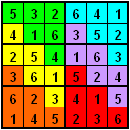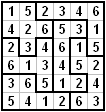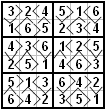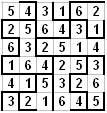## Thursday, 21 January 2010

### Rules for Sudoku Variants

Extra Regions Sudoku
Rules for Extra Regions Sudoku are as follows. In any grid of n numbers,
1-n in each row
1-n in each column
1-n in each block of n
1-n in each diagonal if highlighted
1-n in each coloured set (joined or unjoined)Irregular Sudoku
Rules for Irregular Sudoku are as follows. In any grid of n numbers,

1-n in each row
1-n in each column
1-n in each block of n
1-n in each diagonal if highlightedGreater Than Less Than Sudoku
Rules for Greater Than Less Than Sudoku are as follows. In any grid of n numbers,

1-n in each row
1-n in each column
1-n in each block of n
1-n in each diagonal if highlighted
Arrow always points to smaller valueTorodial Sudoku
Rules for Torodial Sudoku are as follows. In any grid of n numbers,

1-n in each row
1-n in each column
1-n in each block of n (blocks may wrap around to the opposite side of the grid)
1-n in each diagonal if highlighted